# High School Math : How to find the length of the side of a pentagon

## Example Questions

### Example Question #1 : How To Find The Length Of The Side Of A Pentagon

What is the side length of a regular pentagon with a perimeter of?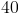Explanation:

To find the side length of a regular pentagon with a perimeter ofyou must use the equation for the perimeter of a pentagon.

The equation is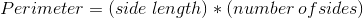Plug in the numbers for perimeter and number of sides to get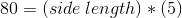Divide each side of the equation by the number of sides to get the answer for the side length.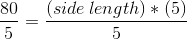The answer is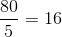.

### Example Question #1 : How To Find The Length Of The Side Of A Pentagon

Find the length of the side of the following pentagon.The perimeter of the pentagon is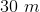.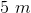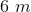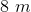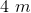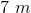Explanation:

The formula for the perimeter of a regular pentagon is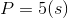,

whererepresents the length of the side.

Plugging in our values, we get: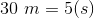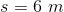### Example Question #3 : How To Find The Length Of The Side Of A Pentagon

Find the length of the side of the following pentagon.The perimeter of the pentagon is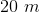.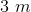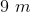Explanation:

The formula for the perimeter of a regular pentagon is,

whererepresents the length of the side.

Plugging in our values, we get: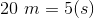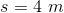### All High School Math Resources